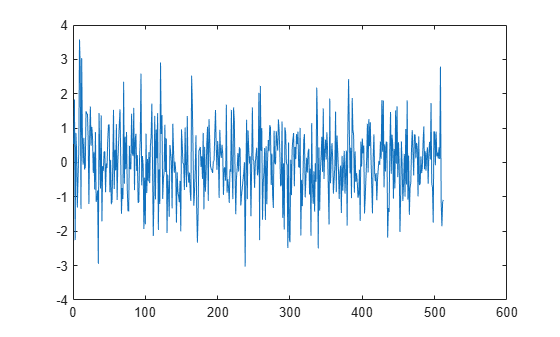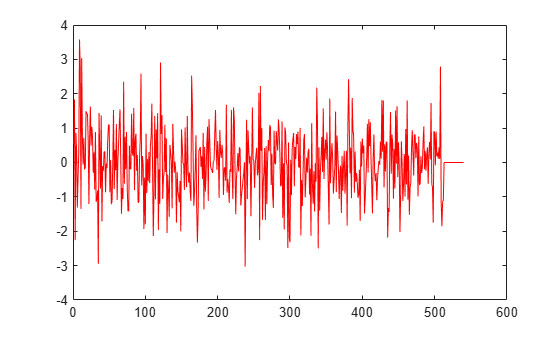# dsp.AsyncBuffer

## Description

The `dsp.AsyncBuffer` System object™ writes samples to and reads samples from a first-in, first-out (FIFO) buffer. The `write` method writes data to the buffer, and the `read` method reads data from the buffer. When creating the object, you can set the number of samples (rows) of the buffer using the `Capacity` property. The number of channels (columns) is set during the first call to `write`. Initialize the buffer by calling `write` or `setup` before the first call to `read`.

The data that you write occupies the next available space in the buffer. If the buffer is full and all the data within it is unread (```asyncBuff.NumUnreadSamples == asyncBuff.Capacity```), the object overwrites the oldest data with any new data that comes in. The buffer removes data only when the data is overwritten, so you can reread data from the past. The `dsp.AsyncBuffer` object supports writing and reading variable frame size signals. For examples, see Read Variable Frame Sizes from Buffer and Write Variable Frame Sizes to Buffer.

To write and read samples from a FIFO buffer:

• Create a `dsp.AsyncBuffer` object and set the properties of the object.

• Call `write` to write samples to the buffer.

• Call `read` to read samples from the buffer.

• Call `peek` to read samples without changing the number of unread samples in the buffer.

## Creation

### Syntax

``asyncBuff = dsp.AsyncBuffer``
``asyncBuff = dsp.AsyncBuffer(cap)``

### Description

example

````asyncBuff = dsp.AsyncBuffer` returns an async buffer System object, `asyncBuff`, using the default properties.```
````asyncBuff = dsp.AsyncBuffer(cap)` sets the `Capacity` property to `cap`.Example: `asyncBuff = dsp.AsyncBuffer(200000);````

## Properties

expand all

Unless otherwise indicated, properties are nontunable, which means you cannot change their values after calling the object. Objects lock when you call them, and the `release` function unlocks them.

If a property is tunable, you can change its value at any time.

Number of writable/readable rows in the buffer, specified as a positive integer greater than or equal to 2. The number of rows during each write to the buffer must not exceed the capacity of the buffer. If the buffer is full and all the data within is unread, the object overwrites the oldest data with any new data that comes in. The `CumulativeOverrun` property returned by `info` gives the number of samples overrun per channel since the last call to `reset`. The number of samples overrun is the number of unread samples overwritten.

By default, this property has data type `int32`.

Example: `asyncBuff = dsp.AsyncBuffer(200000);`

Data Types: `single` | `double` | `int8` | `int16` | `int32` | `int64` | `uint8` | `uint16` | `uint32` | `uint64`

Number of unread samples in each channel (column) of the buffer, specified as an integer greater than or equal to 0. The total number of unread samples in the buffer is `NumUnreadSamples` × numChann. The variable numChann is the number of channels in the buffer. The number of channels in the buffer is the number of data columns in the first call to `write`.

The `CumulativeUnderrun` property returned by the `info` method gives the number of samples underrun per channel since the last call to `reset`. Underrun occurs if you attempt to read more samples than available.

Example: ```asyncBuff = dsp.AsyncBuffer; input = randn(512,1); numUnreadSamples = write(asyncBuff,input)```

Data Types: `int32`

## Usage

To write and read samples from the async buffer:

• Create a `dsp.AsyncBuffer` object and set the properties of the object.

• Call `write` to write samples to the buffer.

• Call `read` to read samples from the buffer.

• Call `peek` to read samples without changing the number of unread samples in the buffer.

## Object Functions

To use an object function, specify the System object as the first input argument. For example, to release system resources of a System object named `obj`, use this syntax:

`release(obj)`

expand all

 `info` Get cumulative overrun and underrun `read` Read data from buffer `write` Write data to buffer `peek` Read data from buffer without changing number of unread samples
 `step` Run System object algorithm `release` Release resources and allow changes to System object property values and input characteristics `reset` Reset internal states of System object

## Examples

expand all

The `dsp.AsyncBuffer` System object™ supports reading variable frame sizes from the buffer.

Create a `dsp.AsyncBuffer` System object. The input is white Gaussian noise with a mean of 0, a standard deviation of 1, and a frame size of 512 samples. Write the input to the buffer using the `write` method.

```asyncBuff = dsp.AsyncBuffer; input = randn(512,1); write(asyncBuff,input); plot(input) hold on```Store the data that is read from the buffer in `outTotal`.

Plot the input signal and data that is read from the buffer in the same plot. Read data from the buffer until all samples are read. In each iteration of the loop, `randi` determines the number of samples to read. Therefore, the signal is read in as a variable-size signal. The `prevIndex` variable keeps track of the previous index value that contains the data.

```outTotal = zeros(size(input)); prevIndex = 0; while asyncBuff.NumUnreadSamples ~= 0 numToRead = randi([1,64]); out = read(asyncBuff,numToRead); outTotal(prevIndex+1:prevIndex+numToRead) = out; prevIndex = prevIndex+numToRead; end plot(outTotal,'r') hold off```Verify that the input data and the data read from the buffer (excluding the underrun samples, if any) are the same. The cumulative number of overrun and underrun samples in the buffer is determined by the `info` function.

`S = info(asyncBuff)`
```S = struct with fields: CumulativeOverrun: 0 CumulativeUnderrun: 28 ```

The `CumulativeUnderrun` field shows the number of samples underrun per channel. Underrun occurs if you attempt to read more samples than available.

Write a sine wave of variable frame size to the buffer. Compute the FFT of the sine wave and visualize the result on an array plot.

Initialize the `dsp.AsyncBuffer`, `dsp.ArrayPlot`, and `dsp.FFT` System objects.

```asynBuff = dsp.AsyncBuffer; plotter = dsp.ArrayPlot; fftObj = dsp.FFT('FFTLengthSource','Property','FFTLength',256); ```

The sine wave is generated using the `sin` function in MATLAB. The `start` and `finish` variables mark the start and finish indices of each frame. If enough data is cached, read from the buffer and perform the FFT. View the FFT on an array plot.

```start = 1; for Iter = 1 : 2000 numToWrite = randi([200,800]); finish = start + numToWrite; inputData = sin(start:finish)'; start = finish + 1; write(asynBuff,inputData); while asynBuff.NumUnreadSamples >= 256 x = read(asynBuff,256); X = abs(fftObj(x)); plotter(log(X)); end end ```Read data from the async buffer without changing the number of unread samples using the `peek` function.

Create a `dsp.AsyncBuffer` System object™. The input is a column vector of 100 samples, 1 to 100. Write the data to the buffer.

`asyncBuff = dsp.AsyncBuffer`
```asyncBuff = AsyncBuffer with properties: Capacity: 192000 NumUnreadSamples: 0 ```
```input = (1:100)'; write(asyncBuff,input);```

Peek at the first three samples. The output is [1 2 3]'.

`out1 = peek(asyncBuff,3)`
```out1 = 3×1 1 2 3 ```

The `NumUnreadSamples` is 100, indicating that the `peek` function has not changed the number of unread samples in the buffer.

`asyncBuff.NumUnreadSamples`
```ans = int32 100 ```

After peeking, read 50 samples using the `read` function. The output is [1:50]'.

`out2 = read(asyncBuff,50)`
```out2 = 50×1 1 2 3 4 5 6 7 8 9 10 ⋮ ```

The `NumUnreadSamples` is 50, indicating that the `read` function has changed the number of unread samples in the buffer.

`asyncBuff.NumUnreadSamples`
```ans = int32 50 ```

Now peek again at the first three samples. The output is [51 52 53]'. Verify that the `NumUnreadSamples` is still 50.

`out3 = peek(asyncBuff,3)`
```out3 = 3×1 51 52 53 ```
`asyncBuff.NumUnreadSamples`
```ans = int32 50 ```

Read 50 samples again. The output now contains the sequence [51:100]'. Verify that `NumUnreadSamples` is 0.

`out4 = read(asyncBuff)`
```out4 = 50×1 51 52 53 54 55 56 57 58 59 60 ⋮ ```
`asyncBuff.NumUnreadSamples`
```ans = int32 0 ```

## Limitations

Before calling the `read` method, you must initialize the buffer by calling either the `write` or `setup` method. For an example, see Why Does the dsp.AsyncBuffer Object Error When You Call read Before write?

Watch now